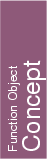## Kernel::ConstructIsoRectangle_2

A model for this must provide:

Kernel::Iso_rectangle_2
 fo.operator() ( Kernel::Point_2 p, Kernel::Point_2 q)
introduces an iso-oriented rectangle with diagonal opposite vertices p and q such that p is the lexicographically smallest point in the rectangle.

Kernel::Iso_rectangle_2
 fo.operator() ( Kernel::Point_2 left, Kernel::Point_2 right, Kernel::Point_2 bottom, Kernel::Point_2 top)
introduces an iso-oriented rectangle fo whose minimal x coordinate is the one of left, the maximal x coordinate is the one of right, the minimal y coordinate is the one of bottom, the maximal y coordinate is the one of top.

### Refines

AdaptableFunctor (with two arguments)